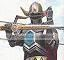-=+=- -=+=- -=+=- -=+=- -=+=- -=+=- -=+=- -=+=- -=+=- -=+=- -=+=- -=+=- -=+=- -=+=- -=+=- -=+=- -=+=- -=+=- -=+=- -=+=- -=+=- -=+=- -=+=- -=+=- -=+=- -=+=- -=+=- -=+=- -=+=- -=+=- (c) WidthPadding Industries 1987 0|123|0 -=+=- -=+=- -=+=- -=+=- -=+=- -=+=- -=+=- -=+=- -=+=- -=+=- -=+=- -=+=- -=+=- -=+=- -=+=- -=+=- -=+=- -=+=- -=+=- -=+=- -=+=- -=+=- -=+=- -=+=- -=+=- -=+=- -=+=- -=+=- -=+=- -=+=- SoCoder -> Article Home -> Graphics 3D How to make great 3D games, from building models and levels, to getting all the collision to work, and more. Older -->Using GLFW with libdispatch on OS X How to properly use GLFW with libdispatch on OS X Cower Monkey MacBump Mapping Bump Mapping in Blitz3D Toaster Blitz WindowsBasic Animation in 3D Studio Max Tutorial A small article I wrote over at syntaxbomb, which I'd like to share here. DabzThe making of Wolfie Night of Hairy Beasts power mousey Older -->